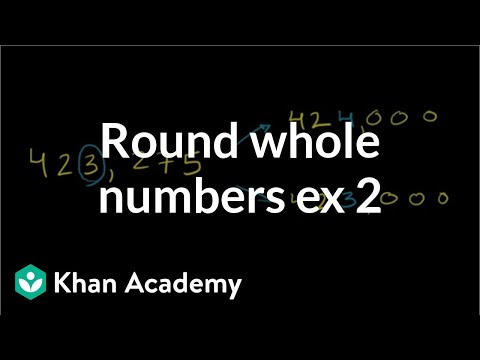Video

# Rounding whole numbers: missing digit (Full video)

Description: Lindsay finds the missing digit in an estimation problem by using a number line. So, we want to know what digits from zero to nine can we fill in here so that this number is closer to 5000 than any other thousand. So if we want to fill in digits here, then we can graph them on our number line and see what are they nearest to. Just, for example, if we plot a number right here, whatever number this point represents, what is its nearest thousand?

### Other videos you might be interested in### Math Studio Talk: Instruction for 4.NBT (Full video)

#### EngageNY